# Quiz 16: Performance Evaluation and Incentive Compensation

Return on investment Return on investments (ROI) is a profitability ratio which is used to determine the profitability of the investment. It also facilitates the comparison of efficiency or profits among different investments. It is calculated by given formula: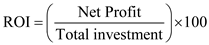Return on assets: Return on assets (ROA) is a financial ratio which is commonly defined as ratio of net income to total assets. It reflects the ability of company of earning income by utilizing assets of company. Here Net income is calculated from the income statement of the company and is considered profit after taxes. It is calculated by given formula: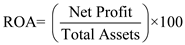Where,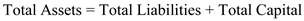Hospital C has invested \$30 million on assets from which \$15 million are firm's own funds and rest \$15 million are borrowed funds. Interest charged on the borrowed sum is 10%. Interest before interest is \$6 million. a. It is required to calculate ROI:Therefore, Rate of return on Investment is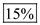Working note: 1. Calculate Net profit: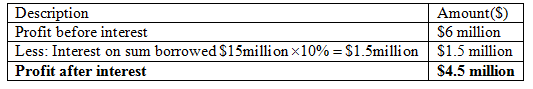Calculate total investment on assets: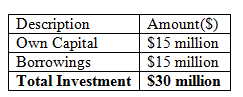b. It is required to calculate Return on assets.Therefore, Rate of return on assets isc. It is required to calculate the Residual Income, if expected rate is 15% Residual Income is an approach in which minimum desired return is defined by health care organization. Here, residual can be understood as an amount which is left after base charge. Higher total residual income represents that management is performing better. It is calculated as: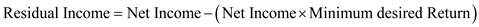Thus, residual income is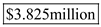d. Net income for next project is \$2.7 million and investment for next projects is \$15 million. Desired return is 15%. It is required to state that which method is better? ROA indicates how efficiently your company generates income using its assets.Therefore, Rate of return on assets is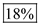ROI, on the other hand, measures profitability in terms of investment.Therefore, Rate of return on Investment isResidual income has advantage over ROI. Base charged used in RI can be applied to whole assets irrespective of facts whether source of assets is equity or debt.Thus, residual income is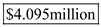So from above calculations it can be concluded that Residual Income method should be used.